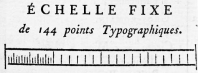'The Fournier scale: two inches in total, divided into four half-inches, the medium intervals are one line (​112inch), and the smallest intervals are ​136 inch; no intervals for the point is given, though Fournier printed a reference scale of 144 points over two inches; however, it was too rough to accurately measure a single point (Wikipedia article on Typographic Unit).A: Paris, Île-de-France, France

# Pierre-Simon Fournier le Jeune Publishes the Point System or Typographic Unit

1737

In 1737 Parisian printer and type-founder Pierre-Simon Fournier le Jeune published Tables des proportions des differens caracteres de l'imprimerie, describing his point-system, or typographic unit for the sizes of type. According to Fournier's system, type sizes were multiples of a unit which he termed a "point typographique" based on a scale of 144 points.

The "Fourier point," was based on a foot, 1 foot equals 12 inches, 1 inch (pouce) was divided into 12 lines (lignes) and 1 line was further divided into 6 typographic points (points typographiques). 1 point Fournier = 0.0135 inches.